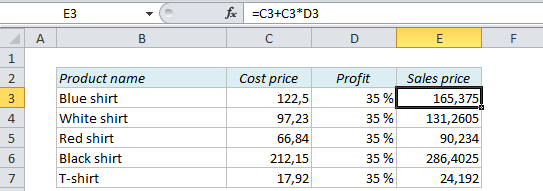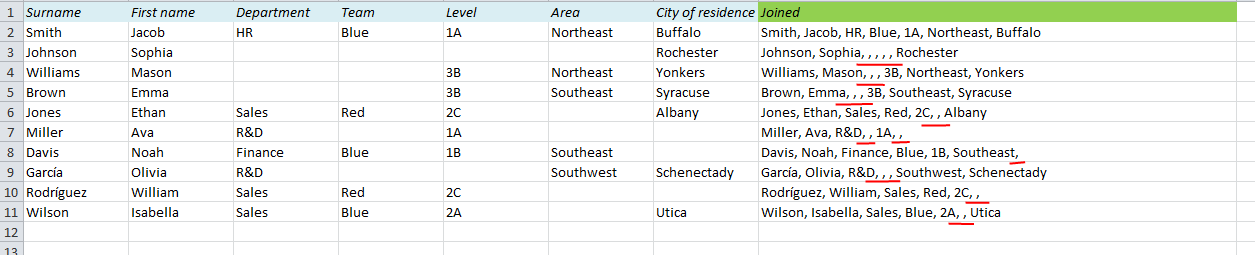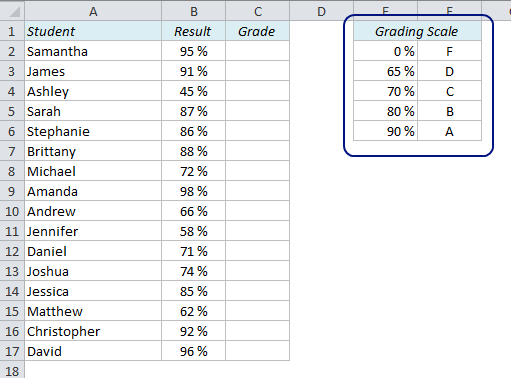# How to calculate prices and make them end with a certain number in Excel

## How to calculate prices and make them end with a certain number in Excel

When you calculate prices you usually know the cost price and the desired profit percentage, so all you have to do is to add the profit to the cost price in order to calculate the sales price. However, the price list might not look as nice as you want:# Join cells in Excel and remove excess commas between elements

Unclean data can cause a lot of problems in Excel. In this post I will show how you can join data from different columns with a comma between them. That’s the easy part. The problem occurs when you have empty cells in your data, like in the table below. The result of the first row looks fine, but if you look at the rows with empty cells, you get too many commas:# How to Calculate Grades in Excel

How can we calculate the grades (A-F) in Excel if we have the test results as numbers? We know that a score of 90% or higher is an A, 80-89% is a B, 70-79% is a C, 65-69% is a D and less than 65% is an F.

The first thing we should do is to organize this information in a lookup table:# Highlight Weekends in Excel with Conditional Formatting

The picture to the right shows a table with some sales figures for July. There’s nothing wrong with the table as it is, but I find it very hard to read and make sense of it. There are just a lot of numbers and dates, and you can’t even distinguish between weekdays and weekends. If we could highlight the weekends (or weekdays) it would be a lot easier to read these numbers. In this example I want to highlight the Saturdays and Sundays. Here’s how we’ll do it:

# How VLOOKUP can get you in trouble and how to solve it

## An Extra Column Means Trouble

If you want to find a value in a table in Excel, a simple VLOOKUP function is usually a good and easy way to do it. But you have to be careful – if you insert a new column in your table, the function might not work anymore, and we have to find another approach. Here’s why: